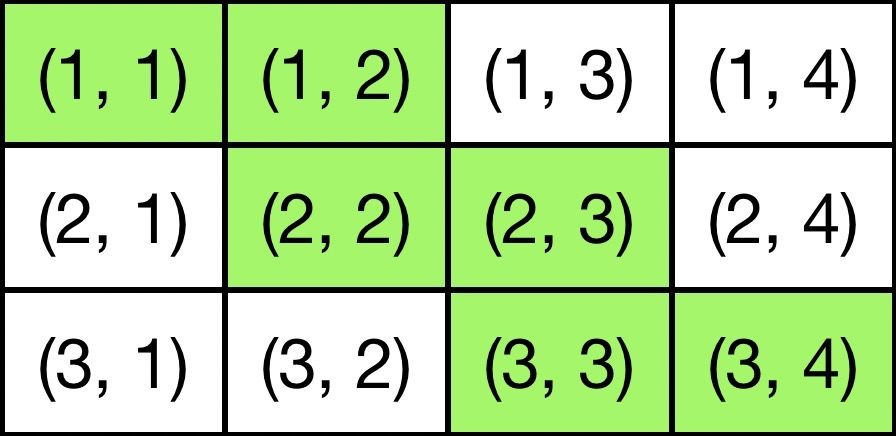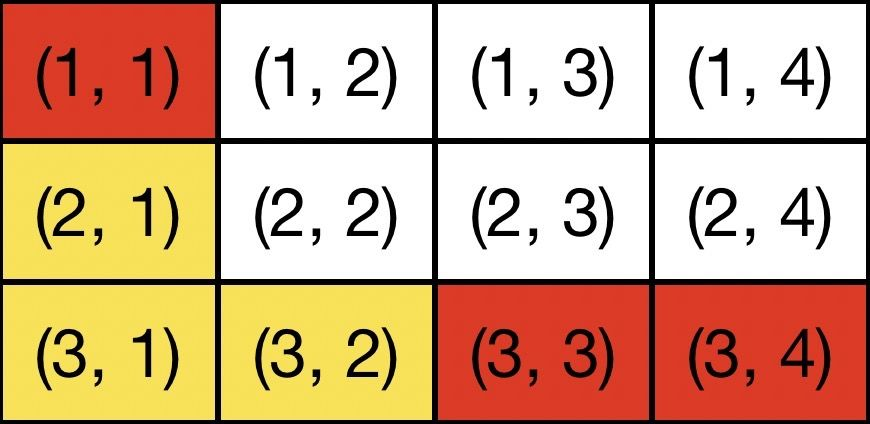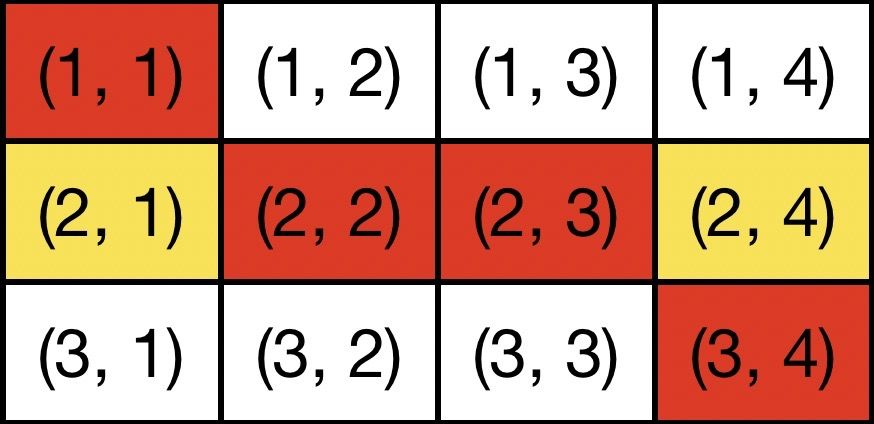시간 제한 메모리 제한 제출 정답 맞은 사람 정답 비율
1 초 512 MB 1 1 1 100.000%

## 문제

You are going to take part in robot programming contest. You will have to launch robots to a special field that has detectors at some cells. The jury has chosen one of the shortest paths on the field and you have to find it.

The field is a grid rectangle with $m$ rows and $n$ columns. Let us denote the cell at the $i$-th row and the $j$-th column as $(i, j)$ ($1 \le i \le m$, $1 \le j \le n$). Every path that the robot can be launched along must start at the top-left cell of the grid which has coordinates $(1, 1)$, pass some cells of the field, and end at the bottom-right cell which has coordinates $(m, n)$.

In one step the robot can move one cell down, or one cell to the right. Therefore if the robot is at the cell $(i, j)$, it can move to the cell $(i + 1, j)$ or to the cell $(i, j + 1)$. The robot cannot move outside of the field. The path of the robot consists of $m + n - 2$ steps, so it moves from the cell $(1, 1)$ to the cell $(m, n)$ visiting some other cells.

The jury has chosen one of the possible robot paths. This path is not known to participants. Each cell that belongs to the path chosen by the jury has special detector installed in it. When the robot enters the cell with the detector, the detector reports that the cell was visited.

The participant can launch the robot along any allowed path from $(1, 1)$ to $(m, n)$. After every launch the jury tells the participant which cells that have detectors installed have reported that the cell was visited. Your task is to launch the robot at most $10$ times, and find out the path that the judges have chosen.

## 프로토콜

First your program receives two integers $m$ and $n$ --- the size of the field for the robot ($1 \le m, n \le 1000$, $m + n > 2$).

After that your program must make queries, each query represents one launch of the robot.

To make a query output "? $s$", where $s$ is the string that denotes the path that the robot must follow. The string must have a length $n+m-2$ and consist of 'D' and 'R' characters. If the $i$-th character is 'D' on the $i$-th step the robot moves down, if the $i$-th character is 'R', it moves to the right. The robot starts its path in a cell $(1, 1)$, and after all steps it must be in the cell $(m, n)$.

As the answer the jury outputs an integer $t$ --- the number of cells with detectors that have reported the robot has passed them ($2 \le t \le m + n - 1$). The following $t$ lines contain two integers $r_i$ and $c_i$ each --- the coordinates of the cells $(r_i, c_i)$ with detectors that have reported the robot has passed them (for all $1 \le i \le t$ the inequalities $1 \le r_i \le m$ and $1 \le c_i \le n$ hold). It is guaranteed that all $t$ cells are distinct. They are printed by increasing of $r_i$, and for equal $r_i$ by increasing of $c_i$.

After you detect the chosen path, your program must print the line "! $s$}" where $s$ is the string that specifies the chosen path in the format described above.

If your program makes more than $10$ queries, makes incorrect query, or answers the path incorrectly, it would get the verdict Wrong Answer.

## 예제 입력 1

3 4

3
1 1
3 3
3 4

4
1 1
2 2
2 3
3 4


## 예제 출력 1


? DDRRR

? DRRRD

! RDRDR


## 힌트

The picture 1 shows the path chosen by the jury in the first sample test. The string that specifies this test is "RDRDR".Picture 1. The chosen path is “RDRDR”.

The picture 2 shows the first query. The cells of the path "DDRRR" are denoted with bold frame. The cells that belong to the path chosen by the jury, the ones that have the detector that reports the presence of the robot, are shaded.

The picture 3 shows the second query. The cells of the path "DRRRD" are denoted with bold frame. The cells that belong to the path chosen by the jury, the ones that have the detector that reports the presence of the robot, are shaded.Picture 2. Query "DDRRR".Picture 3. Query "DRRRD".

## 채점

• 예제는 채점하지 않는다.